### Algebra Class 8th Mathematics Term 2 Tamilnadu Board Solution

##### Question 1.Solve the following equations:3x + 5 = 23Answer:Subtracting 5 from both sides,⇒ 3x + 5 – 5 = 23 – 5⇒ 3x = 18Dividing both sides by 3,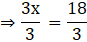⇒ x = 6Question 2.Solve the following equations:17 = 10 - yAnswer:Subtracting 10 from both sides,⇒ 17 - 10 = 10 – y – 10⇒ 7 = -yDividing both sides by -1,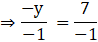⇒ y = -7Question 3.Solve the following equations:2y - 7 = 1Answer:Adding 7 to both sides,⇒ 2y – 7 + 7 = 1 + 7⇒ 2y = 8Dividing both sides by 2,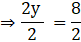⇒ y = 4Question 4.Solve the following equations:6x = 72Answer:Dividing both sides by 6,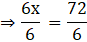⇒ x = 12Question 5.Solve the following equations: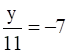Answer:Multiplying both sides by 11,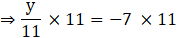⇒ y = -77Question 6.Solve the following equations:3(3x - 7) = 5(2x - 3)Answer:9x – 21 = 10x – 15⇒ 10x – 15 – 9x + 21 = 0⇒ x + 6 = 0⇒ x = -6Question 7.Solve the following equations:4(2x - 3) + 5(3x - 4) = 14Answer:⇒ 8x – 12 + 15x – 20 = 14⇒ 8x + 15x = 14 + 12 + 20⇒ 23x = 46Dividing both sides by 23,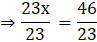⇒ x = 2Question 8.Solve the following equations: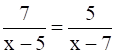Answer:⇒ 7(x – 7) = 5(x – 5)⇒ 7x – 49 = 5x – 25⇒ 7x – 5x = 49 – 25⇒ 2x = 24Dividing both sides by 2,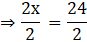⇒ x = 12Question 9.Solve the following equations: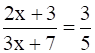Answer:⇒ 5(2x + 3) = 3(3x + 7)⇒ 10x + 15 = 9x + 21⇒ 10x – 9x = 21 – 15⇒ x = 6Question 10.Solve the following equations: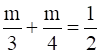Answer: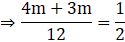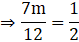⇒ 7m × 2 = 12⇒ 14m = 12Dividing both sides by 2,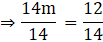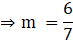Question 11.Frame and solve the equations for the following statements:Half of a certain number added to its one third gives 15. Find the number.Answer:Let the number be xThen according to question,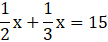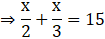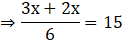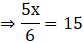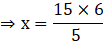⇒ x = 18Hence, the number is 18.Question 12.Frame and solve the equations for the following statements:Sum of three consecutive numbers is 90. Find the numbers.Answer:Let the numbers be x, x+1 and x+2Then according to the question,x + (x + 1) + (x + 2) = 90⇒ x + x + 1 + x + 2 = 90⇒ 3x + 3 = 90⇒ 3x = 90 – 3⇒ 3x = 87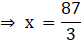⇒ x = 29⇒ x + 1 = 29 + 1 = 30⇒ x + 2 = 29 + 2 = 31Hence, the numbers are 29, 30 and 31.Question 13.Frame and solve the equations for the following statements:The breadth of a rectangle is 8 cm less than its length. If the perimeter is 60 cm, find its length and breadth.Answer:Let breadth of rectangle = xThen length of rectangle = x + 8Perimeter = 60 cmWe know that,Perimeter of rectangle = 2 (length of rectangle + breadth of rectangle)⇒ 60 = 2(x + (x + 8))⇒ 60 = 2(x + x + 8)⇒ 60 = 2(2x + 8)⇒ 60 = 4x + 16⇒ 4x = 60 – 16⇒ 4x = 44⇒ x = 11⇒ x + 8 = 11 + 8 = 19Hence, breadth of rectangle = 11 cmlength of rectangle = 19 cmQuestion 14.Frame and solve the equations for the following statements:Sum of two numbers is 60. The bigger number is 4 times the smaller one. Find the numbers.Answer:Let the smaller number be xThen bigger number = 4xThen according to the question,x + 4x = 60⇒ 5x = 60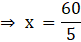⇒ x = 12⇒ 4x = 4 × 12 = 48Hence, the numbers are 12 and 48.Question 15.Frame and solve the equations for the following statements:The sum of the two numbers is 21 and their difference is 3. Find the numbers. (Hint: Let the bigger number be x and smaller number be x – 3)Answer:Let the bigger number be xThen the smaller number = x – 3 [∵ the difference is 3]Then according to the question,x + (x – 3) = 21⇒ 2x – 3 = 21⇒ 2x = 21 + 3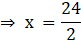⇒ x = 12⇒ x – 3 = 12 – 3 = 9Hence, the numbers are 12 and 9.Question 16.Frame and solve the equations for the following statements:Two numbers are in the ratio 5 : 3. If they differ by 18, what are the numbers?Answer:Let the numbers be 5x and 3xThen according to the question,5x – 3x = 18⇒ 2x = 18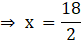⇒ x = 9⇒ 5x = 5 × 9 = 45⇒ 3x = 3 × 9 = 27Hence, the numbers are 45 and 27.Question 17.Frame and solve the equations for the following statements:A number decreased by 5% of it is 3800. What is the number?Answer:Let the number be xThen according to the question,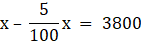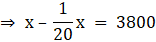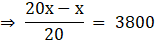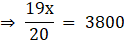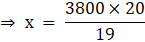⇒ x = 4000Hence, the number is 4000.Question 18.Frame and solve the equations for the following statements:The denominator of a fraction is 2 more than its numerator. If one is added to both the numerator and their denominator the fraction becomes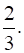Find the fraction.Answer:Let the numerator be xThen the denominator = x + 2Then according to the question,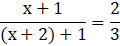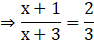⇒ 3(x + 1) = 2(x + 3)⇒ 3x + 3 = 2x + 6⇒ 3x – 2x = 6 – 3⇒ x = 3 (Numerator)⇒ x + 2 = 3 + 2 = 5 (Denominator)Hence, the fraction is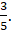Question 19.Frame and solve the equations for the following statements:Mary is 3 times older than Nandhini. After 10 years the sum of their ages will be 80. Find their present ages.Answer:At present,Let age of Nandini = xThen age of Mary = 3xAfter 10 years,Age of Nandini = x + 10Age of Mary = 3x + 10Then according to the question,[x + 10] + [3x + 10] = 80⇒ x + 10 + 3x + 10 = 80⇒ 4x + 20 = 80⇒ 4x = 80 – 20⇒ 4x = 60⇒ x = 15⇒ 3x = 3 × 15 = 45Hence, Present age of Nandini = 15 yearsPresent age of Mary = 45 yearsQuestion 20.Frame and solve the equations for the following statements:Murali gives half of his savings to his wife, two third of the remainder to his son and the remaining ` 50,000 to his daughter. Find the shares of his wife and son.Answer:Let the savings of Murali be x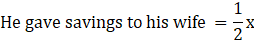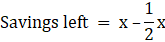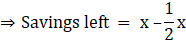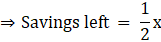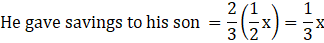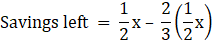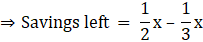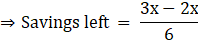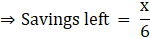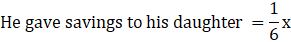According to the question,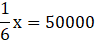⇒ x = 50000 × 6⇒ x = 300000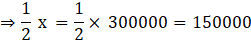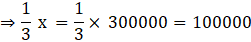Hence, Share of his wife = Rs 1,50,000Share of his son = Rs 1,00,000

PDF FILE TO YOUR EMAIL IMMEDIATELY PURCHASE NOTES & PAPER SOLUTION. @ Rs. 50/- each (GST extra)

HINDI ENTIRE PAPER SOLUTION

MARATHI PAPER SOLUTION

SSC MATHS I PAPER SOLUTION

SSC MATHS II PAPER SOLUTION

SSC SCIENCE I PAPER SOLUTION

SSC SCIENCE II PAPER SOLUTION

SSC ENGLISH PAPER SOLUTION

SSC & HSC ENGLISH WRITING SKILL

HSC ACCOUNTS NOTES

HSC OCM NOTES

HSC ECONOMICS NOTES

HSC SECRETARIAL PRACTICE NOTES

# 2019 Board Paper Solution

HSC ENGLISH SET A 2019 21st February, 2019

HSC ENGLISH SET B 2019 21st February, 2019

HSC ENGLISH SET C 2019 21st February, 2019

HSC ENGLISH SET D 2019 21st February, 2019

SECRETARIAL PRACTICE (S.P) 2019 25th February, 2019

HSC XII PHYSICS 2019 25th February, 2019

CHEMISTRY XII HSC SOLUTION 27th, February, 2019

OCM PAPER SOLUTION 2019 27th, February, 2019

HSC MATHS PAPER SOLUTION COMMERCE, 2nd March, 2019

HSC MATHS PAPER SOLUTION SCIENCE 2nd, March, 2019

SSC ENGLISH STD 10 5TH MARCH, 2019.

HSC XII ACCOUNTS 2019 6th March, 2019

HSC XII BIOLOGY 2019 6TH March, 2019

HSC XII ECONOMICS 9Th March 2019

SSC Maths I March 2019 Solution 10th Standard11th, March, 2019

SSC MATHS II MARCH 2019 SOLUTION 10TH STD.13th March, 2019

SSC SCIENCE I MARCH 2019 SOLUTION 10TH STD. 15th March, 2019.

SSC SCIENCE II MARCH 2019 SOLUTION 10TH STD. 18th March, 2019.

SSC SOCIAL SCIENCE I MARCH 2019 SOLUTION20th March, 2019

SSC SOCIAL SCIENCE II MARCH 2019 SOLUTION, 22nd March, 2019

XII CBSE - BOARD - MARCH - 2019 ENGLISH - QP + SOLUTIONS, 2nd March, 2019

# HSCMaharashtraBoardPapers2020

(Std 12th English Medium)

HSC ECONOMICS MARCH 2020

HSC OCM MARCH 2020

HSC ACCOUNTS MARCH 2020

HSC S.P. MARCH 2020

HSC ENGLISH MARCH 2020

HSC HINDI MARCH 2020

HSC MARATHI MARCH 2020

HSC MATHS MARCH 2020

# SSCMaharashtraBoardPapers2020

(Std 10th English Medium)

English MARCH 2020

HindI MARCH 2020

Hindi (Composite) MARCH 2020

Marathi MARCH 2020

Mathematics (Paper 1) MARCH 2020

Mathematics (Paper 2) MARCH 2020

Sanskrit MARCH 2020

Sanskrit (Composite) MARCH 2020

Science (Paper 1) MARCH 2020

Science (Paper 2)

Geography Model Set 1 2020-2021

MUST REMEMBER THINGS on the day of Exam

Are you prepared? for English Grammar in Board Exam.

Paper Presentation In Board Exam

How to Score Good Marks in SSC Board Exams

Tips To Score More Than 90% Marks In 12th Board Exam

How to write English exams?

How to prepare for board exam when less time is left

How to memorise what you learn for board exam

No. 1 Simple Hack, you can try out, in preparing for Board Exam

How to Study for CBSE Class 10 Board Exams Subject Wise Tips?

JEE Main 2020 Registration Process – Exam Pattern & Important Dates

NEET UG 2020 Registration Process Exam Pattern & Important Dates

How can One Prepare for two Competitive Exams at the same time?

8 Proven Tips to Handle Anxiety before Exams!